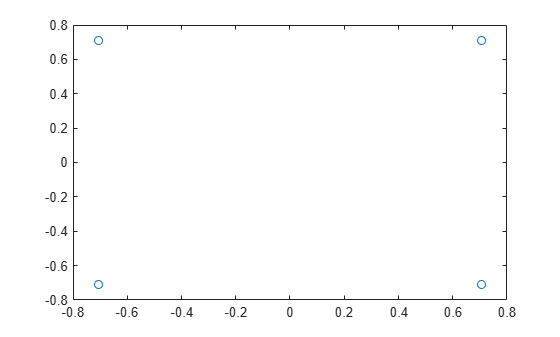# ltePSCCHDRS

PSCCH demodulation reference signal

## Syntax

``````[seq,info] = ltePSCCHDRS``````
``````[seq,info] = ltePSCCHDRS(ue)``````

## Description

example

``````[seq,info] = ltePSCCHDRS``` returns a 24-by-1 complex column vector sequence containing PSCCH demodulation reference signal (DM-RS) values and an associated information structure. For more information, see PSCCH Demodulation Reference Signal Processing.```

example

``````[seq,info] = ltePSCCHDRS(ue)``` returns a vector of DM-RS values for either D2D or V2X sidelink given the specified UE settings structure. For more information, see PSCCH Demodulation Reference Signal Processing.```

## Examples

collapse all

Generate a PSCCH DM-RS sequence associated with both DM-RS SC-FDMA symbols in a subframe. Plot the constellation of the sequence, which is QPSK modulated.

```[pscchDrsSeq,info] = ltePSCCHDRS; plot(pscchDrsSeq,'o')```Generate the PSCCH DM-RS sequence associated with the four DM-RS SC-FDMA symbols of a PRB pair for a PSCCH transmission and V2X sidelink.

Create a user equipment settings structure for the V2X sidelink mode with PRB set indices of 0 and 1 and a zero cyclic shift.

```ue = struct('SidelinkMode','V2X'); ue.PRBSet = [0 1]'; ue.CyclicShift = 0;```

Generate a PSCCH DM-RS sequence associated with both DM-RS SC-FDMA symbols in a subframe. The output sequence is a 96-length vector to be mapped onto the 24 subcarriers in each of the pair of DM-RS SC-FDMA symbols per slot for two consecutive resource blocks.

```[pscchDrsSeq,info] = ltePSCCHDRS(ue); size(pscchDrsSeq)```
```ans = 1×2 96 1 ```

## Input Arguments

collapse all

User equipment settings, specified as a parameter structure containing these fields:

Sidelink mode, specified as `'D2D'` or `'V2X'`.

Data Types: `char` | `string`

Zero-based physical resource block (PRB) index, specified as an integer, an integer column vector, or a two-column integer matrix.

For D2D sidelink, the PSCCH is intended to be transmitted in a single PRB in a subframe and therefore, specifying `PRBSet` as a scalar PRB index is recommended. For V2X sidelink, the PSCCH is intended to be transmitted in a pair of consecutive PRB in a subframe, therefore `PRBSet` must be a column vector containing two consecutive indices. However, for a more general nonstandard multi-PRB allocation, `PRBSet` can be a set of indices specified as an integer column vector or as a two-column integer matrix corresponding to slot-wise resource allocations for PSCCH.

Data Types: `double`

Cyclic shift for DM-RS, specified as `0`, `3`, `6` or `9`. The function uses this input only for V2X sidelink.

Data Types: `double`

Data Types: `struct`

## Output Arguments

collapse all

PSCCH DM-RS values, returned as a complex column vector. For more information, see PSCCH Demodulation Reference Signal Processing.

Data Types: `double`
Complex Number Support: Yes

PSCCH DM-RS information about the intermediate variables used to create the DM-RS, returned as a parameter structure containing these fields:

Reference signal cyclic shift for each slot, returned as a two-column vector. (α)

`Alpha` is proportional to `NCS`, where $\alpha =\frac{2\pi {n}_{cs,\lambda }}{12}$.

Base sequence group number for each slot, returned as a two-column vector. (u)

Base sequence number for each slot, returned as a two-column vector. (v)

Root Zadoff-Chu sequence index for each slot, returned as a two-column vector. (q)

Cyclic shift values for each slot, returned as a two-column vector. (${n}_{\text{cs,}\lambda }$)

Zadoff-Chu sequence length, returned as an integer. (${N}_{ZC}^{RS}$)

Orthogonal cover value for each slot, returned as a matrix. ($\overline{w}$)

Data Types: `struct`

collapse all

### PSCCH Demodulation Reference Signal Processing

The PSCCH demodulation reference signal (DM-RS) sequence is transmitted alongside the `ltePSCCH` values using the two SC-FDMA symbols allocated to DM-RS in a PSCCH subframe. By default, the output vector is the repetition of a 12-element sequence and specified in TS 36.211, Section 9.8. The output vector is mapped onto the 12 subcarriers of the DM-RS SC-FDMA symbol in each slot of the single PSCCH physical resource block (PRB) transmission on antenna port 1000. For a V2X configured PSCCH, the output will be a 96-by-1 vector to be mapped onto the 24 subcarriers in each of the pair of DRS SC-FDMA symbols per slot for two consecutive resource blocks.

The single-PRB PSCCH DM-RS is transmitted using a short base QPSK reference sequence instead of the Zadoff-Chu sequence that is normally used for reference signals. Because the Zadoff-Chu sequence is not used, the `RootSeq` and `NZC` fields are set to –1 in the `info` structure returned by `ltePSCCHDRS`.

### PSCCH Demodulation Reference Signal Indexing

Use the indexing function, `ltePSCCHDRSIndices`, and the corresponding sequence function, `ltePSCCHDRS`, to populate the resource grid for any PSCCH subframe. The PSCCH DM-RS is transmitted in the available SC-FDMA symbols in a PSCCH subframe, using a single layer on antenna port 1000.

The indices are ordered as the PSCCH DM-RS QPSK modulation symbols should be, applying frequency-first mapping. One-based linear indexing is the default return format but you can also generate alternative indexing formats by using the `opts` input.

The resource elements in the last SC-FDMA symbol within a subframe are counted in the mapping process but should not be transmitted. The sidelink-specific SC-FDMA modulation creates the last symbol, which serves as a guard symbol.

For D2D sidelink, in zero-based indexing, the SC-FDMA symbol indices used are {3,10} for normal cyclic prefix and {2,8} for extended cyclic prefix. The same symbols are used by the `ltePUSCHDRSIndices` function. For V2X sidelink, there are four DM-RS SC-FDMA symbols with indices {2,5,8,11}, defined for normal cyclic prefix only.

Note

The indicated symbol indices are based on TS 36.211, Section 9.8. However to align with the LTE Toolbox™ subframe orientation, these indices are expanded from symbol index per slot to symbol index per subframe.

For more information on mapping symbols to the resource element grid, see Resource Grid Indexing.

 3GPP TS 36.211. “Evolved Universal Terrestrial Radio Access (E-UTRA); Physical Channels and Modulation.” 3rd Generation Partnership Project; Technical Specification Group Radio Access Network. URL: https://www.3gpp.org.